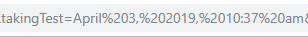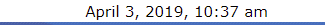# Handling differences in timezones

I created a quiz timer which uses a combo of javascript and PHP. PHP get the quiz’s end time from a URL parameter called `takingTest` and plugs it in the javaScript code. However, it does not work for everyone. It works for everyone if I plug in the value manually. Any ideas what the problem is?

The URL parameterI echoed the URL parameter using `<?php echo \$_GET['takingTest']; ?>` and it showed the correct end time.

Echoed value with PHPWhen it breaks, it returns a minus value and submits the quiz.

Minus value example

-10h -27m -225

My code looks like below:

``````<?php

if((\$_GET["started"]) === "true") {

?>
<!--/*<!-- Quiz countdown block -->

<script type="text/javascript" defer>

\$(function () {

// Set the date we're counting down to
var countDownDate = new Date("<?php echo \$_GET['takingTest']; ?>").getTime();

// Update the count down every 1 second
var x = setInterval(function() {

// Get todays date and time
var now = new Date().getTime();

// Find the distance between now and the count down date
var distance = countDownDate - now;

// Time calculations for days, hours, minutes and seconds
var days = Math.floor(distance / (1000 * 60 * 60 * 24));
var hours = Math.floor((distance % (1000 * 60 * 60 * 24)) / (1000 * 60 * 60));
var minutes = Math.floor((distance % (1000 * 60 * 60)) / (1000 * 60));
var seconds = Math.floor((distance % (1000 * 60)) / 1000);

// Output the result in an element with id="demo"
document.getElementById("timer").innerHTML = hours + "h "
+ minutes + "m " + seconds + "s ";

// If the count down is over, write some text
if (distance < 0) {
clearInterval(x);
}
}, 1000);

});

</script>

<?php } ?>

**Date Generation**

\$defaultTimeZone = 'UTC';
if ( date_default_timezone_get() != \$defaultTimeZone )date_default_timezone_set( \$defaultTimeZone );

// somewhere in the code
function _date( \$format = "r", \$timestamp = false, \$timezone = false ) {
\$userTimezone = new DateTimeZone( !empty( \$timezone ) ? \$timezone : 'GMT' );
\$gmtTimezone = new DateTimeZone( 'GMT' );
\$myDateTime = new DateTime( ( \$timestamp != false ? date( "r", ( int )\$timestamp ) : date( "r" ) ), \$gmtTimezone );
\$offset = \$userTimezone->getOffset( \$myDateTime );
return date( \$format, ( \$timestamp != false ? ( int )\$timestamp : \$myDateTime->format( 'U' ) ) + \$offset );
}

\$time = new DateTime();

\$stamp = \$time->format( "F j, Y, g:i a" );

\$_SESSION[ "EnddTime" ] = \$stamp;``````

Can you give an example of what date/time is breaking the code?

``````\$_SESSION[ "EnddTime" ] = \$stamp;
``````

Is this a proper way to set a session variable in JavaScript? I couldn’t see where you’d come back out to PHP. And should that be “EndTime” with a single “d”? May be irrelevant, I don’t know where you use that value.

The session had already been set somewhere else. I did that when I was trying to figure out what was going on. it returns a minus value for some people and grades their quiz while they haven’t even started.

Really need to see what date/time values are being used, and step through the code to see where it’s going wrong. You show the `_date()` function definition, but I don’t see where it’s being used.

Ignore the `\$_SESSION` comment, I wasn’t reading it properly.

Did you scroll through my post? Maybe, I do not understand your question. Sorry about that.

I thought I had, might be missing something though.

Is the client in the same timezone as the server?

users are in different time zones.

On the face of it I can’t see anything wrong, maybe you could add a bit of code to log the date / time value that is causing the negative calculations.

Does it go negative part-way through the test, or will it always fail at the very start?

Instantly

Suggesting a problem in where the date/time is generated, rather than any of the JS code.

Beyond me, sorry.

Force the use of UTC across the board instead.

I.e. the date/time you pass along to the JS side should be in UTC, and you should also convert the users date/time to UTC before continuing with the script.

Edit:
Or, pass along the time they have available for the test, and then append the time to their local date/time to get the expire/submit time.

Note. In either case remember to store the expire/submit time on the server as well, since JS can be edited at any time to extend the timer…

I am not that familiar with date handling in either language, but I thought the OP was forcing UTC in both sides - certainly the JS new date function seems to default to creating a UTC time, and I’d presumed that the idea of the `_date()` function was to achieve the same thing, and figured that the switching between UTC and GMT literals in there would be OK.

But yes, just passing back the duration and having the JS work with that would probably simplify things a lot.

### Beware:

If your PHP and JavaScript functions are returning the “number of seconds since Jan 1st 1970” Note that the former returns seconds while the latter returns milliSeconds.

Edit:
The following tests worked fine when the milliSeconds were converted to seconds:

``````echo jj. \$x=1554988416286;
echo jj .'JS new Date().getTime() ==> 1554988416286';
echo jj .'JS new Date().getTime() ==> ' .date('F jS, Y, H:i a', \$x);

echo jj. \$x=1554988416;
echo jj .'JS new Date().getTime() ==> 1554988416';
echo jj .'JS new Date().getTime() ==> ' .date('F jS, Y, H:i a', \$x);

echo '<h4> display the number of milliseconds since midnight, January 1, 1970.
</h4>';

<br>
### Output:
UTC
PHP now ==> 1554989402
April 11th, 2019, 13:30 pm

PHP (int) microtime(true) ==> 1554989402
April 11th, 2019, 13:30 pm

PHP now ==> 1554989402
April 11th, 2019, 13:30 pm

1554988416286
JS new Date().getTime() ==> 1554988416286
JS new Date().getTime() ==> August 15th, 51245, 02:44 am

1554988416
JS new Date().getTime() ==> 1554988416
JS new Date().getTime() ==> April 11th, 2019, 13:13 pm

display the number of milliseconds since midnight, January 1, 1970.``````

Please, how exactly do I do that?

How do I apply this to my case?

It is now late here so perhaps another user could supply the necessary script to get you started.

Okay, thank you!

As I read it (which I proved was “not very well”, the first time, by not noticing it had exited back to PHP for the end bit…) both of the conversions to seconds are being done on the JavaScript end. The formatted date and time is output by PHP into the JS code, which then creates a new Date() from it.

This topic was automatically closed 91 days after the last reply. New replies are no longer allowed.